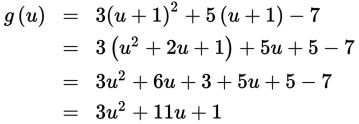# SAT Math Multiple Choice Question 255: Answer and Explanation

### Test Information

Question: 255

15. If g is a function defined over the set of all real numbers and g(x - 1) = 3x2 + 5x - 7, then which of the following defines g(x)?

• A. g(x) = 3x2 - x - 9
• B. g(x) = 3x2 + 5x + 1
• C. g(x) = 3x2 + 11x + 1
• D. g(x) = 3x2 + 11x - 6

Explanation:

C

Difficulty: Hard

Category: Passport to Advanced Math / Functions

Strategic Advice: The key to answering this question is in having a conceptual understanding of function notation. Here, the input (x - 1) has already been substituted and simplified in the given function. Your job is to determine what the function would have looked like had x been the input.

Getting to the Answer: To keep things organized, let u = x - 1, the old input. This means x = u + 1. Substitute this into g and simplify:This means g(u) = 3u2 + 11u + 1.

When working with function notation, you evaluate the function by substituting a given input value for the variable in the parentheses. Here, if the input value is x, then g(x) = 3x2 + 11x + 1.